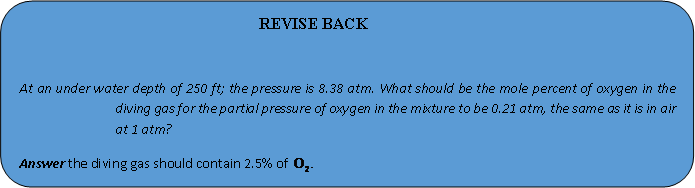# DALTON’S LAW OF PARTIAL PRESSURE

## DALTON’S LAW OF PARTIAL PRESSURE

According to this law total pressure of a non reacting mixture of gases is equal to the sum of partial pressures of individual gases.

P = PA + PB + PC + ……………….. (in mixture of gases which do not react chemically)

Let nA moles of A, nB moles of B and nC moles of C are filled in a vessel of volume V maintained at temperature T. Then, total pressure will be

PV = (nA + nB + nC)RT (Gas equation) …..(i)

Let only nA moles of A is added in the vessel then

PAV = nART …..(ii)

Similarly,

PBV = nBRT …..(iii)

PCV = nCRT …..(iv)

On adding, (ii), (iii) and (iv) we get

(PA + PB + PC)V = (nA + nB + nC) RT …..(v)

Now comparing equation (i) and (v) we getAlternatively

Dividing equation (ii) by (i)= XA (mole fraction of A)

PA = PXA …..(i)

Since, PB = PXB …..(ii)

PC = PXC …..(iv)

on adding (i), (ii) and (iii) we get

(PA + PB + PC) = P (XA + XB + XC)

Similarly, XA  + XB + XC =1

So,The law can even be applicable to a mixture of reacting gases under the condition when they have attain equilibrium (at which the number of moles of each constituent species remains fixed.

Example6   A 2.5 litre flask contains 0.25 mole each of sulphur dioxide and nitrogen gas at 27°C. Calculate the partial pressure exerted by each gas and also the total pressure.

Solution: Partial pressure of SO2= 2.49 × 105 Nm–2 = 2.49 × 105 Pa

Similarly= 2.49 × 105 Pa

Following Dalton’s Law

P Total =+= 2.49 × 105 Pa + 2.49 × 105 Pa = 4.98 × 105 Pa